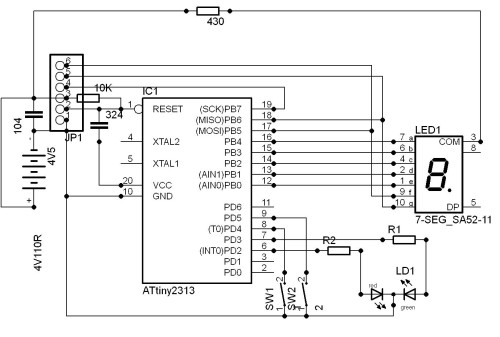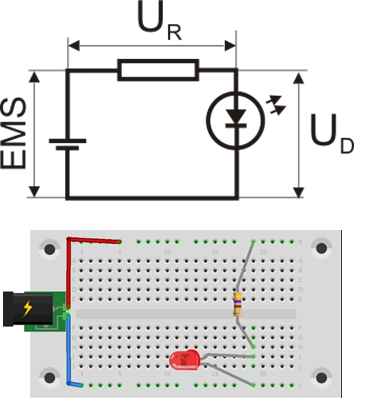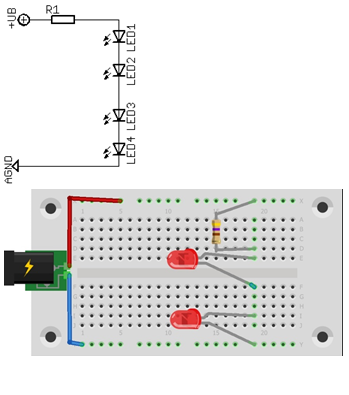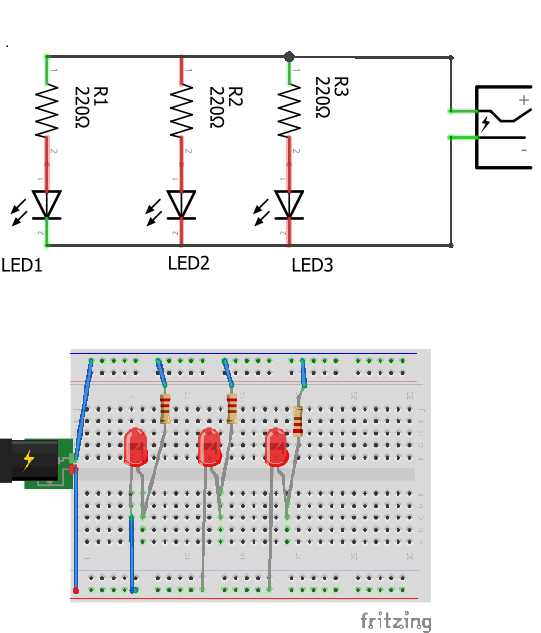This free e-book was created withIt's simple and free.

# Basic Electronic Exercises• Joined Dec 2017
• Published Books 1

How to startup LED

```LED (Light Emiting Diode) is a semiconductor element
that emits light at the current flow. It's a current
consumer and you have to keep it in mind. It is not
important the voltage to which you are connecting
but the current flowing through is.

How to limit current, ie calculate serial resistance:
If the EMS is source voltage,UR is voltage drop on
the resistor and UD is a voltage drop on the diode.
EMS = UR + UD UR = EMS - UD the current is constant
so it can be written as: I × R = EMS - UD ...
and finally what we need is the value of resistance:
R = (EMS - UD)/I  A Practical Example:
We want to connect a red LED to a 5V (USB output
on a computer) R = (5V -2V)/ 0,02A R =150 Ohm.
It is not recommended to use the LEd diode at full
power. Most of them were based on germanium so that
the peak temperature was 90 ° C. Insert the 220 Ohm
resistor and you will not even notice the difference
in the intensity of the light. I = (EMS -UD) / R
(do not run away from Ohm's law :)) I = (5 - 2)/ 220,
I = 0,014A ili I=14mA It works great and very long.```
1Serial conection of LEDs and resistor

```The most used LE diodes made for a current up to 20mA, which means
that the serial resistor should be dimensioned to limit the current
below the limit value, as I already said "less than 20mA".
Characteristics of individual LED you will find on the net,
so-called Datasheet. The voltage drop depends on the type and color
of the LED. In principle it is about 1.5 to 4V.```
```For example: Standard1
20 mA LEDs (ranging from approximately 40 mW to 90 mW) at around:
1.9 to 2.1 V for red, orange, yellow, and traditional green
3.0 to 3.4 V for pure green and blue
2.9 to 4.2 V for violet, pink, purple and white```
`1.https://en.wikipedia.org/wiki/Light-emitting_diode`
3

Serial connection of multiple LEDs

```If we want to connect multiple LEDs to the same source we can do it
in several ways:
a) serial connection
b) a parallel connection
c) a combined connection```
Serial connection of two or more LEDs
```EMS = UR + ∑UD
If you use LEDs of the same type they are:```
```EMS = UR + n×UD , where n is number of LEDs in serial conection.
R1 = (EMS - n×UD) / I
R1 = (12V - 4×2V)/0,02A
R1 = (12-8V)/0,02A
R1= 200 Ohma
We used the first major, 220 Ohm. visit page:
How much energy is released on this resistor: Typically, this is a
small amount of heat that can withstand the most commonly used 1/4
Watt resistor. But check it out: P = U × I; P = 0.02 × 4V
P = 0.08W.```
` `
4###### Parallel connection of multiple LEDs
```If we want to connect multiple LEDs in parallel then each of them
must have their resistor, otherwise we will have uneven glow or
even some will not light up in general. The parallel connection is
only considered if the drive voltage is low because the energy
losses here are very high.```
Example: The resistance calculation is the same as in the first example
R = (EMS – UD) / I
If we connect to 5V
R = (5V -2V) / 0.02A
R = 150 Ohms.
R1 = R2 = R3 …
6This free e-book was created withSkip to content